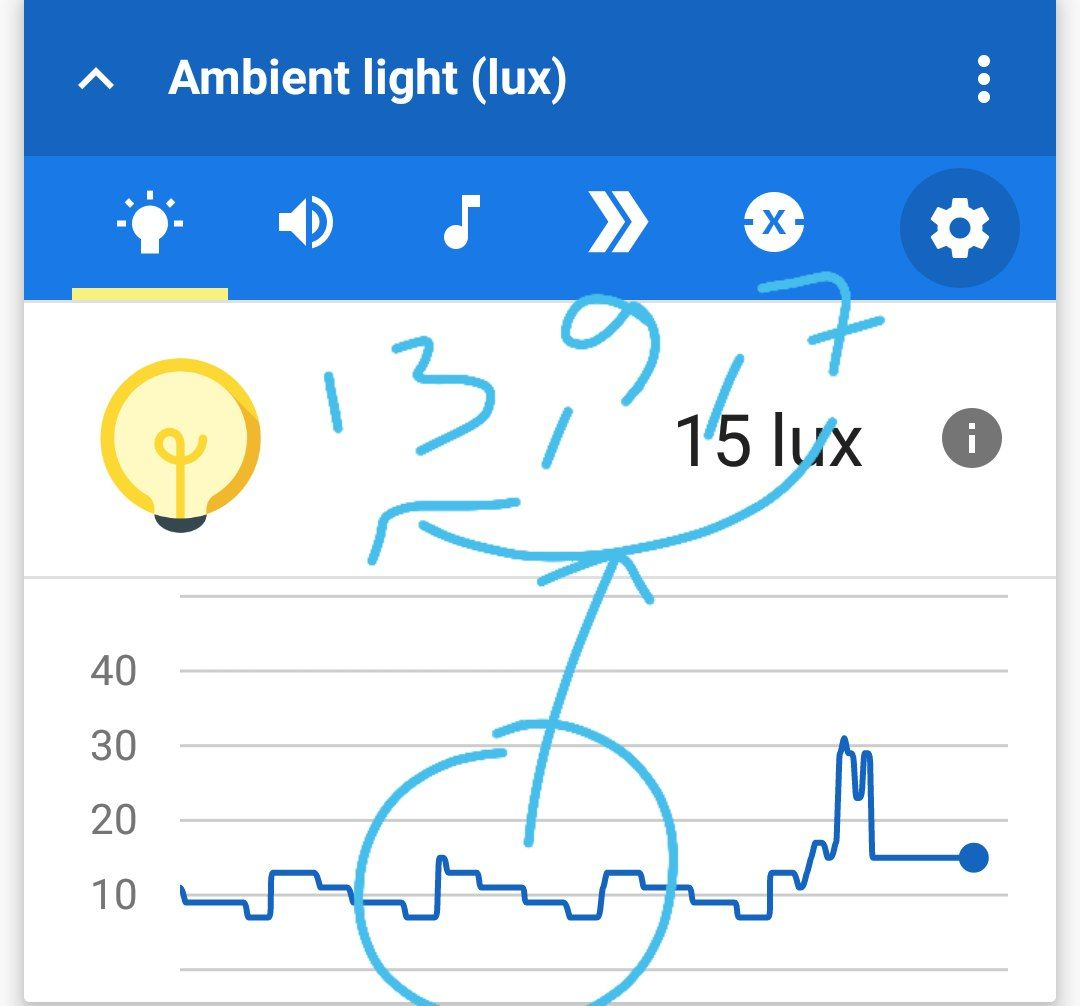# Intensity change by varying the on_time , off_time of inbuilt-LED on Arduino

https://youtu.be/TdAcSeBjhWk

So we discussed strategies to imitate PWM effect by changing on-time/off-time of the led on arduino. Keeping the on-time as 20 milliseconds and gradually decreasing it produces the smooth transition from high intensity to low intensity. (The total time on+off remains constant)

Thereafter, I placed the smartphone sensor to capture the light intensity value during this transition. Ofcourse, its not a controlled experiments, but this initial tinkering led to some observations which are exciting! This provides a context to discuss many concepts including data handling, persistence of vision etc… Here is the graph of the same,The graph shows pattern and the intensity fluctuation between 7-13 lux

Here is the code:

``````
void setup() {
pinMode(13, OUTPUT);
Serial.begin(9600);
}
int iVal=20;
int on_time=iVal;

void loop() {
if(on_time>0){
for (int count=0; count<10;count++){
digitalWrite(13, HIGH);
delay(on_time);
digitalWrite(13, LOW);
delay(iVal-on_time);
}
on_time=on_time-1;
}
else{
on_time=iVal;
}
}

``````

Do share what do you think are some interesting questions to explore/experiments to design and your observations when you attempted to do something similar

5 Likes

Add a tact switch. By pressing the switch increase the brightness.
Then add one more tact switch. Pressing this one should reduce brightness.

Now instead of pin 13 only simultaneously turn on or pin 13 and 12.
On pin 12 connect a resistor of 1K. Connect the other end to +ve of 1uf capacitor and another resistor of 4k7. Connect other end of 1uf capacitor and resistor to gnd. Now measure the voltage on the +ve pin of capacitor. You should get a higher voltage everytime you press increment and a lower voltage everytime you press decrement. You now have a crude Digital to Analog converter.

The intensity measurement with phone sensor is excellent.

3 Likes

Here is some code for PWM with a button press controlled decrement.
Notice the use of delayMicroseconds() and change of the count from 20 to 4096.
Everyone take shot at explaining why this is being done.
"
int SW = 3;
int SWState;
int PwmVal=4096;
int PwmValoff;

void setup()
{
pinMode(13, OUTPUT);
pinMode(SW, INPUT);
Serial.begin(9600);
}

void loop()
{
//Serial.println(“Voidloop”);
//Serial.println(PwmValoff);
//Serial.println(PwmVal);
//digitalWrite(13, HIGH);
//delay(PwmVal);
//digitalWrite(13, LOW);
//delay(PwmValoff);
Pwm_Val();
Pwm_Func(PwmVal, PwmValoff);
}

int Pwm_Func (int, int)
{
//Serial.println(“Pwm_Func”);
//Serial.println(PwmValoff);
//Serial.println(PwmVal);
digitalWrite(13, HIGH);
delayMicroseconds(PwmVal);
digitalWrite(13, LOW);
delayMicroseconds(PwmValoff);
}

int Pwm_Val()
//Serial.println(“Pwm_Val”);
//Serial.println(SWState);
//Serial.println(PwmVal);
if (SWState == LOW)
{ PwmVal=PwmVal-1;
//Serial.println(PwmVal);
PwmValoff=4096-PwmVal;
//Serial.println(PwmValoff);
//Serial.println(PwmVal);
//delay(1000);
if (PwmVal== 0)
{ PwmVal=4096;
}
}
return (PwmVal, PwmValoff);
}
"

1 Like

One can use a multimeter to get an average voltage reading. The voltage changes smoothly from 0 to 4.8 volts. So does the LED intensity.
This is now a single bit Digital to Analog Converter.

2 Likes

PWM with increment and decrement buttons.

"int SWdec = 3; //input for reducing brightness
int SWinc = 2; //input for reducing brightness
int SWState; //state of pin 3
int PwmIncDec; // set +1 if SWinc is pressed or set +1 if Swdec is pressed or 0 if both are high or low
int PwmVal=4096; //max value of on+offtime in u sec
int PwmValon; //on time of Pwm
int PwmValoff; // off time Pwm

void setup()
{
pinMode(13, OUTPUT); //set pin 13 to output - using onboard LED
pinMode(SWdec, INPUT); //set pin 3 to input for key press
pinMode(SWinc, INPUT); //set pin 2 to input for key press
Serial.begin(9600);
}

void loop()
{
//Serial.println(“Voidloop”);
//Serial.println(PwmValoff);
//Serial.println(PwmVal);
//digitalWrite(13, HIGH);
//delay(PwmVal);
//digitalWrite(13, LOW);
//delay(PwmValoff);
Pwm_Val();
Pwm_Func(PwmValon, PwmValoff);
}

/function for setting pin 13 high/low as per value returned from function Pwm_Val/

int Pwm_Func (int, int)
{
//Serial.println(“Pwm_Func”);
//Serial.println(PwmValoff);
//Serial.println(PwmVal);
digitalWrite(13, HIGH);
delayMicroseconds(PwmValon);
digitalWrite(13, LOW);
delayMicroseconds(PwmValoff);
}

/* Check input pin if low decremet PwmVal by 1. If high do not decrement.

• If PwmVal=0 reset PwmVal to 4096. Return /
/
int Pwm_Val()
{ PwmIncDec = 0;
}
{ PwmIncDec = 0;
}
{ PwmIncDec = 1;
}
{ PwmIncDec = -1;
}
//Serial.println(“Pwm_Val”);
//Serial.println(SWState);
//Serial.println(PwmVal);
//if (SWState == LOW)
{ PwmValon=PwmValon-PwmIncDec;
//Serial.println(PwmVal);
PwmValoff=PwmVal-PwmValon;
//Serial.println(PwmValon);
//Serial.println(PwmValoff);
//delay(1000);
if (PwmValon<= 0)
{ PwmValon=PwmVal;
}
else if (PwmValon >= 4096)
{ PwmValon=0;
}
}
return (PwmValon, PwmValoff);
}"
1 Like